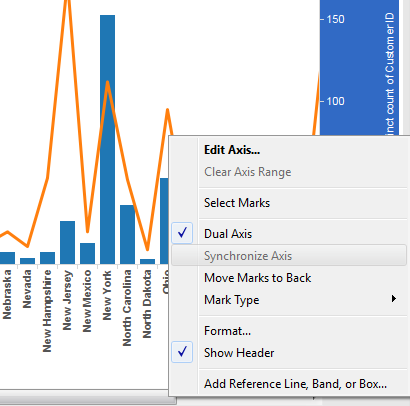# Tableau - Scenario 9 *Sometimes we will see synchronize axis option as disabled. why? And how to solve this? )

Problem: Some times we will see synchronize axis option as disabled. why? And how to solve this?

Description:

In this chart I am showing State wise Sales on one axis and on another axis I am showing countD of Order ID.I am using Dual axis for this chart. When I am trying to Synchronize both axis, I am seeing
Synchronize axis option as disabled. See belowWhy I am Seeing Synchronize Axis Option Disabled?

Ans: When you are trying to Dual axis two different types of measuresI mean two different data types of measures then we will see Synchronize Axis option as disabled.

In this example I am using Sales and CountD(Order ID). Sales numbers have floating values. Where asCountD(Order ID) will give us Integer value.

For State New York:

Sum(Sales): \$839,593.73 (Which is float number)
CountD(Order ID): 402 (Which is Integer)

So if you come across this kind of scenario you wont see Synchronize Axis Option.

How to solve this?

Solution:

Solution is very simple. You need to convert integer values into float.
Create a calculate field and use that field in report.

float(CountD(Order ID))

Create dual axis chart using Sales and New calculated field. Now you will see Synchronize Axis Option Enabled.